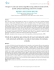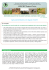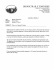PDF file

Transcription

PDF file
```Click
Here
WATER RESOURCES RESEARCH, VOL. 42, W08421, doi:10.1029/2005WR004398, 2006
for
Full
Article
Uncertainty quantification of satellite precipitation estimation
and Monte Carlo assessment of the error propagation
into hydrologic response
Yang Hong,1,2 Kuo-lin Hsu,1 Hamid Moradkhani,1 and Soroosh Sorooshian1
Received 30 June 2005; revised 9 March 2006; accepted 28 March 2006; published 12 August 2006.
 The aim of this paper is to foster the development of an end-to-end uncertainty
analysis framework that can quantify satellite-based precipitation estimation error
characteristics and to assess the influence of the error propagation into hydrological
simulation. First, the error associated with the satellite-based precipitation estimates is
assumed as a nonlinear function of rainfall space-time integration scale, rain intensity, and
sampling frequency. Parameters of this function are determined by using high-resolution
satellite-based precipitation estimates and gauge-corrected radar rainfall data over the
southwestern United States. Parameter sensitivity analysis at 16 selected 5 5 latitudelongitude grids shows about 12–16% of variance of each parameter with respect to its
mean value. Afterward, the influence of precipitation estimation error on the uncertainty of
hydrological response is further examined with Monte Carlo simulation. By this approach,
100 ensemble members of precipitation data are generated, as forcing input to a conceptual
rainfall-runoff hydrologic model, and the resulting uncertainty in the streamflow
prediction is quantified. Case studies are demonstrated over the Leaf River basin in
Mississippi. Compared with conventional procedure, i.e., precipitation estimation error as
fixed ratio of rain rates, the proposed framework provides more realistic quantification of
precipitation estimation error and offers improved uncertainty assessment of the error
propagation into hydrologic simulation. Further study shows that the radar rainfallgenerated streamflow sequences are consistently contained by the uncertainty bound of
satellite rainfall generated streamflow at the 95% confidence interval.
Citation: Hong, Y., K.-L. Hsu, H. Moradkhani, and S. Sorooshian (2006), Uncertainty quantification of satellite precipitation
estimation and Monte Carlo assessment of the error propagation into hydrologic response, Water Resour. Res., 42, W08421,
doi:10.1029/2005WR004398.
1. Introduction
 Precipitation is a key forcing variable to the land
surface hydrological process at all space-timescales. Therefore a better understanding of the spatial and temporal
distribution of precipitation is critical to water resources
management where the sizes of basins range from 100 km2
to 100,000 km2 and the temporal integration of rainfall
inputs ranges from hours to days. However, precipitation
observation from ground-based radar or gauge measurement
for the remote areas and mountain regions is very limited.
Recent development of satellite-based precipitation-retrieval
techniques has been providing extended precipitation coverage beyond ground in situ data [Adler et al., 2003; Ba and
Gruber, 2001; Bellerby et al., 2000; Hong et al., 2004,
2005; Hsu et al., 1997; Huffman et al., 2001; Joyce et
al., 2004; Kuligowski, 2002; Kidd et al., 2003; Miller et al.,
1
Center for Hydrometeorology and Remote Sensing, Department of Civil
and Environmental Engineering, University of California, Irvine, California, USA.
2
Now at NASA Goddard Space Flight Center, Greenbelt, Maryland,
USA.
Copyright 2006 by the American Geophysical Union.
0043-1397/06/2005WR004398\$09.00
2001; Sorooshian et al., 2000; Xie et al., 2003]. While these
algorithms provide precipitation products at spatial and
temporal scales relevant to atmospheric and hydrological
applications, quantification of the estimation error associated
with the products at a range of space scales and timescales
is critical due to the emerging use of satellite precipitation
products in the study of hydrologic and water management
applications. A great deal of progress in evaluating rainfall
[Anagnostou et al., 1999; Carpenter and Georgakakos,
2004; Ciach et al., 2003; Huffman, 1997; Hossain et al.,
2004; Hossain and Anagnostou, 2004; Krajewski et
al., 1991, 2000; Krzysztofowicz, 1999; Li et al., 1996,
1998; McCollum and Krajewski, 1998; Rudolf, 1993;
Steiner et al., 1999; Steiner, 1996; Steiner et al., 2003].
In one of those studies, Huffman  developed a
statistical relationship of the root-mean-square random
errors associated with precipitation estimates. Ciach and
Krajewski  proposed the error variance separation
(EVS) method to decompose the uncertainty of reference
error into retrieval error and sampling error. Gebremichael
et al.  assessed the key factors, such as gauge density
and sampling size, that affect the accuracy of EVS results.
Steiner et al.  proposed a framework to identify
possible relationships between sampling error in radar
W08421
1 of 15
W08421
HONG ET AL.: ERROR PROPAGATION FROM SATELLITE RAINFALL
Figure 1. A conceptual ‘‘end-to-end’’ error analysis framework for precipitation data producers and
users.
Figure 2. (a) Geographic domain and satellite-based versus radar-based accumulated monthly rainfall
over all selected boxes in (b) August 2003 and (c) June 2004; and (d-e) the occurrence of monthly rainfall
for the 2 months. Note that the S/R is the ratio of satellite versus radar rainfall, Corr is correlation
coefficient, and RMSE is the root-mean-square error.
2 of 15
W08421
HONG ET AL.: ERROR PROPAGATION FROM SATELLITE RAINFALL
W08421
W08421
^
Table 1. Reference Error s/R(%)
at a Range of Spatiotemporal
Scales at Box M in June 2004a
1 hour
3 hours
6 hours
12 hours
24 hours
0.04
0.12
0.24
0.48
0.96
1.1269
0.9457
0.8761
0.8316
0.7866
1.065
0.925
0.871
0.833
0.785
0.984
0.867
0.825
0.790
0.758
0.839157
0.813293
0.741898
0.732958
0.703407
0.782346
0.735735
0.698290
0.692426
0.675744
a
See Figure 2a.
rainfall estimates and several other factors. Hossain et al.
 conducted a sensitivity analysis to understand the
implication of satellite Passive Microwave (PM) rainfall
retrieval and sampling error on flood prediction uncertainty
for medium-sized (100 km2) watersheds. In a follow-up
paper, Hossain and Anagnostou  examined the
passive microwave (PM)- and infrared (IR)-based satellite
rainfall retrieval for flood prediction using a probabilistic
error model. Hossain and Anagnostou  also explored
the uncertainty bound of simulated flood events based on
various microwave rainfall samples and hourly infraredbased rainfall estimates. The above research indicated that
it is critical to improve the communication between precipitation data producer and user community by quantifying the estimation error and assessing the influence of error
propagation into hydrologic processes. For data providers,
the error inherent in the retrieval algorithm is of particular
interest. However, from the perspective of data users, the
error associated with the data and the influence of the error
propagation into the hydrologic responses is generally
interesting. As shown in Figure 1, quantification of error
associated with precipitation products would provide more
useful information for users (decision makers) than no error
assessment at all. To quantify the influence of estimation
error on hydrologic responses by ensemble simulation
would further place an appropriate degree of confidence
from data users’ perspectives.
 This study aims to foster the development of an endto-end uncertainty propagation analysis framework that can
quantify the error of satellite rainfall estimation and further
assess the uncertainty of the error propagation into various
hydrological modeling and applications. The error is
expressed as a nonlinear multivariate function of space-time
integration scale, rain intensity, and sampling frequency.
The advantage of this error formula, for example, is that the
various users of the precipitation products can approximate
the error by simply implementing the function at their
specified space-timescales and rain intensities. The influence of precipitation estimation error on a conceptual
rainfall-runoff hydrologic model is examined through
Table 2. Number of Grids for the Statistical Computation at
Box M in June 2004a
1 hour
3 hours
6 hours
12 hours
24 hours
0.04
0.12
0.24
0.48
0.96
10929571
3665026
1839258
931103
462603
1172898
392174
196388
99003
48789
278442
93145
46657
23529
11598
68965
23048
11539
5838
2869
16787
5524
2733
1341
666
Figure 3. Satellite-based rainfall estimation error distribution as a function of spatial and temporal resolution without
consideration of rain intensity.
Monte Carlo simulation. By this approach, an ensemble of
precipitation data is generated, as forcing to the hydrologic
model, and the resulting uncertainty in the hydrologic
response is quantified at different confidence intervals.
 The outline of this paper is as follows: The formulation
of precipitation estimation error is described in dection 2, and
the data used in the study and the calibration procedure to
approximate the error function are presented in section 3. The
uncertainty of streamflow prediction associated with the
satellite rainfall estimation error is assessed in section 4,
and the summary and discussion are given in section 5.
2. Error Quantification Framework
 There is a fundamental difference between satellite and
radar remotely sensed observations of precipitation and those
measured directly by rain gauges. While satellites and radar
measure rainfall as a space integral at a given instance in time,
rain gauges, on the other hand, measure rainfall as a time
integral of rain at a particular location. Let r(x, t) represent the
rain rate (mm h1) at a point x and in time t. Then, the rainfall
at point x accumulated during time period T is
RT ðxÞ ¼
1
T
Z
T
rðx; t Þdt
ð1Þ
0
and the instantaneous rain rate (at time t) averaged over an
area of A is
R A ðt Þ ¼
1
A
Z
A
rðx; t Þdx
ð2Þ
0
The RA(t) is of interest for satellite rainfall estimation because
it is the typical measurement by the satellite at each visit.
Equation (2) also indicates the nature of the measurements
from satellite data: rainfall is the quantity measured as an
integral of space (A) at a point in time (t). Integration of the
instantaneous rain rate r(x, t), both in time and space, gives the
space-time average of rain rate
RAT ¼
1
AT
Z
0
T
Z
0
A
rðx; tÞdxdt ¼
1
T
Z
0
T
RA ðtÞdt ¼
1
A
Z
A
RT ðxÞdx
0
ð3Þ
a
See Figure 2a.
3 of 15
HONG ET AL.: ERROR PROPAGATION FROM SATELLITE RAINFALL
W08421
W08421
^ (100%) at Various Spatialtemporal Scales and 10 Bins of Incremental Rain Ratea
Table 3. Reference Error s/R
Rain Rate, mm/h
Spatial, deg
0.1 – 0.5
0.5 – 1
1–2
2–3
3–4
4–5
5 – 10
10 – 20
>20
0.04
0.12
0.24
0.48
0.96
553.4
370.2
263
233.2
99.13
283.5
225.8
197.5
138.7
105.4
189.3
156.4
131
105.5
79.04
139.3
127.3
106.9
93.07
71.11
1 Hour
111.8
104.7
98.45
85.2
72.25
103.1485
101.2412
91.29212
77.3654
71.28587
99.5772
93.03398
86.49507
70.61353
70.26871
93.0648
90.108
83.5329
70.8495
65.4098
83.686
82.135
79.156
69.504
-
0.04
0.12
0.24
0.48
0.96
295.2
229.4
168.6
151.4
89.1
180.2
152.6
127.8
102.8
85.39
125
109.8
105.6
89.69
75.58
102.1
98.03
91.78
82.03
72.87
3 Hours
93.73
93.1
85.09
71.48
70.26
90.52022
85.17111
80.34503
67.73598
64.23749
86.95734
81.61962
75.57973
70.19385
60.69096
79.7471
78.5683
73.4305
71.4969
54.8749
75.879
73.791
73.036
-
0.04
0.12
0.24
0.48
0.96
200.2
171.5
133.3
111.1
84.86
130
118.7
110.9
91.63
82.8
101.6
96.97
90.09
82.66
69.49
92.46
88.98
83.9
74.61
60.4
6 Hours
86.46
79.59
75.61
68.58
55.8
83.27407
77.38816
71.0505
65.31336
56.77127
77.91234
75.14635
69.18679
64.8041
-
70.7205
70.5529
68.9132
62.5515
-
74.324
-
0.04
0.12
0.24
0.48
0.96
167.4
143.5
106.9
89.76
80.11
109.3
102.9
93.22
86.07
73.26
94.83
88.29
83.55
75.1
61.08
83.59
77.19
73.8
69.58
61.75
12 Hours
75.16
74.95
74.29
59.19
56.31
72.53024
71.64718
64.39437
56.75801
-
69.59589
65.70086
59.66018
-
69.583
60.075
-
-
0.04
0.12
0.24
0.48
0.96
121.4
105.8
93.4
80.64
78.08
94.71
87.64
83.85
71.41
70.31
82.94
78.46
70.42
67.77
59.11
71.38
71.14
68.2
61.1
58.72
24 Hours
65.25
69.36
68.24
-
65.19843
-
-
-
-
a
Dash indicates no data.
Equation (3) assumes perfect measurement of rainfall over an
area A and time period T. Because of the imperfect nature of
satellite rainfall estimation algorithms, there is always an
error associated with the retrieval of the RA(t). Here, we
^ A(t). Supposing
denote the rain rate averaged over area A as R
that the satellite makes N visits over an area A during a time
period T, one can write the satellite-derived rainfall at spacetime averaged scale as
comparison with independent reference data (Rref
AT, e.g.,
radar or gauge rainfall). The reference error is often used to
approximate the true error (e) (see Appendix A for an
explanation). Both the standard deviation and the variance
are measures of spread of the reference error (e). The
standard deviation is the square root of the variance and has
the desirable property of being in the same units as the data.
That is, in the case of this study, both rainfall rate and its
error standard deviation are in mm h1:
T
=Dt
N
X
X
^ AT ¼ 1
^ A ðti Þ ¼ Dt
^ ðt i Þ
R
R
R
N i¼1
T i¼1 A
where Dt(= T/N) is the time interval between consecutive
visits, which is also called sampling frequency. The error
^ AT can be written as
associated with R
N
1 X
^ ðti Þ RAT
R
N i¼1 A
T
Z T
=Dt
Dt X
^ A ðt i Þ 1
¼
RA ðtÞdt
R
T i¼1
T 0
vﬃﬃﬃﬃﬃﬃﬃﬃﬃﬃﬃﬃﬃﬃﬃﬃﬃﬃﬃﬃﬃﬃﬃﬃﬃﬃﬃﬃﬃﬃﬃﬃﬃﬃﬃﬃﬃﬃﬃﬃﬃﬃﬃﬃﬃﬃﬃﬃﬃﬃﬃﬃﬃﬃ
!
qﬃﬃﬃﬃﬃﬃﬃﬃﬃﬃﬃﬃﬃﬃﬃﬃﬃﬃﬃﬃﬃﬃﬃﬃﬃﬃﬃﬃﬃﬃﬃﬃ
ﬃ u
T =Dt
u
Dt X ^
t
ref
ref
^
sE ¼ Var RAT RAT ¼ Var
RA ðti Þ RAT
ð6Þ
T i¼1
ð4Þ
^ AT RAT ¼
e¼R
ð5Þ
The error of precipitation estimate, sE, is a function of its
area coverage (A), time integration (T), sampling frequency
^ inversely
(Dt), and the space-time average of rain rate (R),
proportional to A and T and nonlinearly proportional to Dt
^ In this study, similar to Steiner
(sampling frequency) and R.
et al. , the area A is substituted with spatial scale L,
the side length of A. Thus the nonlinear multivariate
function random error, sE, is written as
In reality, there are no ‘‘true data’’ available. The
approximation of the ‘‘true error e’’ can be assessed through
4 of 15
sE ¼ f
1 Dt ^
; ;R
L T
b c
1
Dt ^ d
R
¼a
L
T
ð7Þ
HONG ET AL.: ERROR PROPAGATION FROM SATELLITE RAINFALL
W08421
W08421
Figure 4. (left) Error distribution as a function of spatial and temporal scales at increasing rain rates for
box I (August 2003). (right) Percentage of error to rain rate.
where a, b, c, and d are the parameters of the approximation
^ AT are degrees of
function, and the units of L, T, and R
1
longitude, hour, and mm h , respectively. The sampling
frequency Dt is 1 hour from the satellite observation in this
study. In order to make it comparable to results obtained
from different climate regimes and various seasons, e.g., dry
or wet, the error sE needs to be tied to a rain rate. As shown
in equation (8), the error could also be expressed as
percentage (%) of rain rate by dividing the space-time
^
average of rain rate (R):
b c
Dt ^ d1
^ ¼ f 1 ; Dt ; R
^ ¼a 1
R
sE =R
L T
L
T
ð8Þ
3. Data and Analysis Procedure
3.1. Data
 The satellite rainfall estimates used in this study
are from the PERSIANN-CCS (Precipitation Estimation
from Remote Sensed Information using Artificial Neural
Network– Cloud Classification System) algorithm [Hong et
al., 2004]. The PERSIANN-CCS is the follow-up version of
our earlier reported work, the PERSIANN algorithm [Hsu et
al., 1997; Sorooshian et al., 2000]. The PERSIANN-CCS is
a patch-based cloud classification and rainfall estimation
system based on coregistered passive microwave and infrared images from low Earth-orbiting and geostationary
satellites by using computer image processing and pattern
recognition techniques. We operate this system (http://hydis8.
eng.uci.edu/CCS/) with the goal of producing data at spatialtemporal resolution (reaching 4 4 km2 30 min) suitable
for basin-scale hydrological research and applications.
PERSIANN-CCS has been generating precipitation estimates at resolution (0.04 0.04 scale and 30-min time
interval) since 2000.
 The National Weather Service WSR-88D stage IV
data serve as ground reference rainfall data at 4 km 4 km
spatial and hourly temporal resolution. Both stage II and
5 of 15
HONG ET AL.: ERROR PROPAGATION FROM SATELLITE RAINFALL
W08421
Table 4. Parameter Estimation for Each of the 16 Selected Boxes
Boxes
a
b
c
d
RMSE, %
A
B
C
D
E
F
G
H
I
J
K
L
M
N
O
P
All
Maximum
Minimum
Range
Mean
Median
STD
Variance
1.11
0.9794
0.8791
0.7279
0.842
0.9755
0.9451
0.9241
0.8674
0.7842
0.9274
0.9659
0.7211
0.9703
0.7492
0.6854
0.8018
1.1100
0.6854
0.4246
0.8732
0.8791
0.1166
0.0136
0.2608
0.2751
0.2274
0.193
0.3051
0.2205
0.2865
0.192
0.1962
0.294
0.1774
0.2577
0.2136
0.3406
0.3115
0.1986
0.2317
0.3400
0.1774
0.1632
0.2460
0.2317
0.0496
0.0025
0.328
0.3423
0.3447
0.3103
0.3724
0.2878
0.3538
0.2593
0.2635
0.3613
0.2154
0.325
0.3309
0.4078
0.4288
0.3159
0.3465
0.4288
0.2154
0.2135
0.3290
0.3309
0.0529
0.0028
0.682595
0.696877
0.549421
0.515032
0.727115
0.642486
0.708498
0.613987
0.618213
0.715968
0.965651
0.795838
0.5356
0.762369
0.633527
0.52057
0.5576
0.9657
0.5150
0.4506
0.6618
0.6425
0.1161
0.0135
14.0912
10.57958
17.07047
12.9689
12.99048
15.42445
13.80694
16.25296
14.82851
13.69446
10.66329
10.00643
15.76622
14.0912
16.54722
13.58956
9.5
17.0100
9.5000
7.5705
13.6395
13.8069
2.3190
5.3780
stage IV analysis are national products generated at National
Center for Environmental Prediction (NCEP) from multisensor (radar and gauge) hourly/6 hourly rainfall data on
local 4-km polar stereographic grids. The stage IV data
differ from the NCEP stage II data because the NCEP stage
II data contain no manual quality control (QC), while the
stage IV data benefit from manual QC performed at the
W08421
12 River Forecasting Centers over the continental United
States. The period of stage IV record begins 1 January 2002,
while stage II began to be archived at NCAR on 1 May
1996. The stage IV high spatial and temporal resolution
rainfall analysis provides data useful for testing of satellite
the NCEP stage IV analysis can be found at http://
wwwt.emc.ncep.noaa.gov/mmb/ylin/pcpanl/stage4/.
 The selected study coverage, shown in Figure 2a as
the shaded area, was divided into 16 boxes, 5 5 for
each. Two heavy rainfall seasons (August 2003 and June
2004) were selected for the analysis of uncertainty sE. The
scatterplots of PERSIANN-CCS and radar monthly accumulation rainfall (0.04 grid) during August 2003 and
June 2004, respectively, are shown in Figures 2b and 2c.
Both months show high spatial correlation (Corr) and
relatively low root-mean-square error (RMSE); however,
PERSIANN-CCS underestimates the two summer months
with satellite-to-radar ratio (S/R) of 0.94 and 0.96, respectively. The occurrence of the rainfall for the two months,
respectively, is displayed in Figures 2d and 2e. The
peaks of occurrence indicate that both months received
relatively heavy rainfall over large areas. In August 2003,
the PERSIANN-CCS estimates match the radar at low
rainfall regions (less than 100 mm) but overestimates
rainfall at medium rainfall (100 – 200 mm) and heavy
rainfall regions (>230 mm). In June 2004, false alarms
occur at no rain or light rainfall regions and slightly
underestimate at heavy rainfall regions (>350 mm).
3.2. Case Studies
 Given their processed basic resolution (0.04 0.04
and hourly), both the satellite and radar data sets were
aggregated into a range of discrete temporal (1, 3, 6, 12, and
Figure 5. (a) Estimated parameters in equation (7) from the data of each of the selected boxes (A-P) and
the data of all the boxes (all) (see Figure 2a and Table 4 for detail) and (b) box plot of the four parameters.
6 of 15
W08421
HONG ET AL.: ERROR PROPAGATION FROM SATELLITE RAINFALL
W08421
Figure 6. Plot of the error distribution with respect to L, T, and R using the optimal parameters from
equation (9).
24 hours) and spatial (0.04, 0.12, 0.24, 0.48, and 0.96)
scales. Therefore each box is divided into subgrids with side
lengths of 0.04, 0.12, 0.24, 0.48, and 0.96 latitudelongitude; each subgrid holds rainfall intensities at time
resolutions of 1, 3, 6, 12, and 24 hour for this analysis. The
error analysis is conducted for each box separately. As an
example, box I (August 2003) and box M (June 2004), as
shown in Figure 2a, were selected to calculate the reference
error. Note that the sampling frequency Dt is 1 hour from
the PERSIANN-CCS data.
3.2.1. Calculating the Reference Error as a Function
of Spatial and Temporal Scales
 Regardless of the rainfall intensities, Table 1 shows
^
the reference error s/R(%)
as a function of spatial and
temporal scales at box M, the Rio Grande basin, in June
2004 wet season. The count of effective subgrid numbers
from this statistical computation is listed in Table 2. The
maximum count of a subgrid number at 1-hour 0.04 scale
is 11,250,000 (125 rows 125 columns 24 hour 30 day) within each 5 grid for rainfall data in June 2003.
Similarly, the reference error (%) distribution, calculated at
box I in August 2003, as a discrete function of spatial and
temporal scales is shown in Figure 3. Without consideration
of the rain intensity, Table 1 and Figure 3 show that the
satellite rainfall estimation reference error (%) is a function
of spatial and temporal scales where higher spatial and
temporal resolution is subject to larger reference error.
7 of 15
W08421
HONG ET AL.: ERROR PROPAGATION FROM SATELLITE RAINFALL
W08421
Figure 7. Same as Figure 6, except the error is expressed as percentage (%) of rain rates.
3.2.2. Calculating the Reference Error as a Function
of Spatial-Temporal Scales and Rainfall Intensities
 The satellite rainfall estimates were incrementally
divided into 10 bins according to their intensities; the reference errors are then calculated for each rain rate bin at various
spatial and temporal scales. The reference error, derived from
box M in June 2004, as a function of both spatial-temporal
scales and rainfall intensities is given in Table 3.
 The reference error (sE) distribution at the discrete
temporal and spatial scales and increasing rain rates for box I
in August 2003 are displayed in Figure 4 (left). The same
reference error is shown in Figure 4 (right), but as a percent^
age of rainfall estimates sE/R(%).
 The procedure was repeated for the other 14 grids in
Figure 2a. A reference error look-up table, similar to Table 3,
is established by using the whole year data (July 2003 to
June 2004) for each grid shown in Figure 2a. All the results
(not shown here) support the assumption that the error in
satellite-based rainfall estimation is inversely proportional
to the area and time interval integration and proportional to
the rainfall rate.
3.3. Regression Parameter Estimation
 For each box shown in Figure 2a, we built up a
reference error look-up table from an entire year data set at
various spatial-temporal scales and 10 bins of incremental
rain intensities. By defining the minimum satellite sampling
frequency as 1 hour (Dt = 1), the parameters (a, b, c, and d)
of equation (7) were calibrated using the error look-up table
derived from the 1-year data set for every box. The resulting
parameter estimates of equation (7) for each of the 16 grids
8 of 15
HONG ET AL.: ERROR PROPAGATION FROM SATELLITE RAINFALL
W08421
Figure 8. Study area for streamflow uncertainty estimation associated with rainfall uncertainty.
(Figure 2a) and for the data from all of the boxes are listed
in Table 4. Using the RMSE as calibration criterion for
equation (7), the optimal parameter sets are estimated
separately for the data in each 5 5 grid box (see
Figure 2a). As shown in Figure 5a, the error estimation
function with the optimal parameter set is
sE ¼ f
1 Dt ^
; ;R
L T
0:2317 0:3465
0:5576
1
1
^
¼ 0:8018
R
L
T
ð9Þ
W08421
 The box plots of the calibrated parameters are given in
Figure 5b. The lower and upper lines of the box are the 25th
and 75th percentiles of the sample. The distance between the
top and bottom of the box is the interquartile range. The cross
the line in the middle of the box represents the mean. If the
mean is not centered in the box, the distribution is skewed.
The statistical information of the 17 sets of parameters is also
listed in the bottom rows of Table 4. Figure 5 and Table 4 show
the spatial variability of each parameter derived from different
5 5 latitude-longitude grids. In particular for parameters
b, c, and d, the ranges of values are more than 50% of their
mean values, which shows that the error of satellite-derived
precipitation estimates can be region-dependent. Notably, it is
reasonable to expect that the error in wet season is generally
larger than dry season. For example in box M, the error
simulated from the parameter set in Table 4 is generally
smaller than the error in Table 3. The difference essentially
originates from the fact that the parameters in Table 4 are
calibrated by entire year data , whereas Table 3
represents the error in wet season only (June 2004). Additionally, the parameter fitting is influenced by the uneven
distribution of the rainfall intensity, more heavy rainfall
occurrence in wet season than dry season.
 Using the calibrated optimal parameter set in equation (9), Figure 6 plots the reference error estimates (sE)
with respect to spatial-temporal scales and rainfall intensities; the same reference error estimates but as a percentage
^ are plotted in Figure 7. Note that
of rainfall intensity (sE/R)
in the current study, we arguably assume that the effect of
sampling frequency in equation (7) is negligible because the
PERSIANN-CCS has relatively high sampling frequency
(30-min), one of the advantages of rainfall estimates using
multiple satellites.
4. Propagation of Rainfall Estimation Error
Into Hydrological Response
 As the key forcing variable of hydrological processes,
the precipitation is largely responsible for the variability in
Figure 9. Hydrologic Model (HyMOD) after Boyle et al. .
9 of 15
W08421
HONG ET AL.: ERROR PROPAGATION FROM SATELLITE RAINFALL
W08421
Figure 10. Given the size of the Leaf River basin and the temporal resolution (daily in this case), the
reference error (%) is a function of rain rates according to the error equation (9): (a) the error estimation
using the 17 parameter sets listed in Table 4 and (b) same but the error expressed as percentage of rain
rates. Note that the straight bold line in Figure 10b indicates the fixed reference error as 50% of rain rates.
model outputs. Clearly, evaluation of the error associated
with precipitation products into model behavior is an
indispensable element of improving hydrologic modeling
and data assimilation.
 In the current study, the influence of the error of
input-forcing data, i.e., precipitation, through a conceptual
rainfall-runoff hydrologic model to output forecasting
uncertainty is evaluated by propagating the approximated
PERSIANN-CCS rainfall error estimates with a Monte
Carlo simulation approach. This approach generates an
ensemble of precipitation data as forcing input to fit to the
conceptual rainfall-runoff model, and the resulting uncertainty in the forecasted streamflow is then quantified. The
applicability and usefulness of this procedure is demonstrated
in the case of the Leaf River basin, located north of Collins,
Mississippi. The size of the Leaf River basin is about
1949 km2. A map of the Leaf River basin is shown in
Figure 8. We have used a daily time step for precipitation
input. This may be larger than desirable to capture the
hydrologic response. See, e.g., Burges  for a perspective on this issue.
 To demonstrate rainfall error propagation through a
hydrologic model, we employed a parsimonious conceptual
Hydrologic Model (HyMOD) as described by Boyle et al.
 (Figure 9) in the model simulation. HyMOD has its
origins in the probability-distributed moisture model (PDM)
[Moore, 1985], an extension of some of the lumped storage
models developed in 1960s, and later to the case of multiple
storages representing a spatial distribution of different
storage capacities in a watershed. HyMOD is a rainfall
excess model defined by a nonlinear tank (representative of
the watershed soil moisture content) connected with two
series of linear tanks, three identical quick flow tanks
(responsible for channel routing) in parallel to a slow flow
tank representing the base flow. The model contains five
states of variables describing the dynamics of the system
along with five parameters which characterize the system
 Once the spatial size (1949 km2) and the temporal
resolution (daily in this case) of the Leaf River basin are
specified, the standard error of rainfall as a function of rain
rate can be estimated from equation (9). Figure 10 shows
the error, in terms of RMSE and relative percentage error, of
precipitation based on the regression parameters of those
16 test grid boxes and the averaged parameter set listed in
Table 4. As shown in Figure 10a, the RMSE of estimated
rainfall is increased with respect to rainfall with higher
intensity. In terms of percentage error of estimated precipitation (see Figure 10b), high-intensity rainfall is subjected
to lower-percentage relative error. The straight bold line
shown in Figure 10b assumes the error as fixed ratio (50%)
10 of 15
W08421
HONG ET AL.: ERROR PROPAGATION FROM SATELLITE RAINFALL
W08421
Figure 11. (a) Time series of the daily rainfall over the Leaf River basin; (b) the rainfall estimation error
calculated from equation (9); and (c) rainfall error expressed as percentage of rain rates. Note that the
straight line in Figure 11c indicates rainfall estimation error as fixed percentage of rainfall intensities, and
the bar represents the rainfall error percentage from equation (9).
of rain rates, which is about the average of the rainfall
intensity-dependent error, while the true error property of
rainfall estimates is unknown. The rain intensity-dependent
error reveals more realistic error characteristics of the
rainfall data than the fixed ratio method, one of the
conventional approaches.
 HyMOD streamflow simulation proceeded under
two scenarios: (1) the error as a variable ratio of rain rates
based on equation (9), and (2) the error as a fixed ratio of
rain rates. The satellite daily rainfall estimates over the Leaf
River basin for a time period over 1 year are shown in
Figure 11a. The daily rainfall error estimated from the scaledependent function equation (9) is listed in Figure 11b, and
the percentage of error to the rain rates is given in Figure
11c. In addition, a fixed ratio of 50% of the daily rain rate
error (horizontal line) is also listed as a reference. In each
error scenario, 100 ensemble members of the HyMOD
simulation were used to derive the confidence interval of
streamflow prediction by using the Monte Carol method.
The simulated runoff output and its 95% uncertainty
bound derived from the second scenario are displayed in
Figure 12a; the results from the first scenario are given in
Figure 12b. The red dots represent the runoff time series
generated from the satellite rainfall estimates. The results
show that the reliability of estimated streamflow is highly
related to the intensity of input-forcing data. For both of the
error propagation cases, the uncertainty bound is signifi-
cantly higher during high-flow periods and is lower in lowflow regions. However, the 95% uncertainty bound of the
simulated runoff as shown in Figure 12b is lower than the
one generated from the fixed error ratio case pictured in
Figure 12a. The first scenario, scale-dependent error propagation, offers more realistic uncertainty assessment of
streamflow prediction than the fixed ratio error propagation,
particularly during high flow, i.e., heavy rainfall periods.
 Comparison of runoff simulation generated from
both stage IV radar precipitation measurement and satellite
PERSIANN-CCS measurement is shown in Figure 13.
Again, 100 ensemble members were generated from the
satellite-based rainfall to derive the streamflow responses.
The result of the 66% confidence interval of generated
streamflow is listed in Figure 13a, while the 95% confidence interval is listed in Figure 13b. The red dots represent
the streamflow sequence generated from radar rainfall,
while the solid line shows the synthetic mean of ensemble
streamflows simulated from satellite rainfall estimates. At
the 66% confidence interval, part of the radar-generated
streamflow are not covered by the uncertainty bound, while
under the 95% confidence interval, the radar-generated
streamflow are consistently contained by the uncertainty
bound of satellite-simulated flow. However, the uncertainty
bound for the high flow period can be large, as the
upper bound can be 100% 300% higher than the radar-
11 of 15
W08421
HONG ET AL.: ERROR PROPAGATION FROM SATELLITE RAINFALL
W08421
Figure 12. Uncertainty estimation of streamflow prediction corresponding to 95 percentile confidence
using rainfall error propagation (a) fixed rainfall error 50% and (b) scale-dependent rainfall error
according to equation (9). The red dots are mean of the 100 ensemble streamflow sequences simulated
from satellite rainfall estimates.
generated streamflow values, while the lower bound can
reach to the amount of no streamflow.
5. Summary and Conclusion
 As satellite-based precipitation estimates are increasingly applied to atmospheric and hydrological applications at
various space-timescales, the estimation error itself and the
effect of how the error propagates through models need to be
evaluated as a whole so that the users have a certain degree of
confidence while making decisions (Figure 1). Additionally,
evaluation of error associated with precipitation products and
its propagation into model behavior is an indispensable
element of evaluating data quality and improving hydrologic
simulation techniques. Given these requirements, the aim of
this paper is to foster the development of an end-to-end
analysis framework that can quantify satellite-based precipitation estimation error and assess its influence on the uncertainty of hydrologic simulation.
 Satellite-based rainfall estimates from PERSIANNCCS [Hong et al., 2004] were used in this study.
PERSIANN-CCS, a high-resolution precipitation estimation
system operating at near real-time mode (http://hydis8.eng.
uci.edu/CCS/), has been generating precipitation data at
12 of 15
W08421
HONG ET AL.: ERROR PROPAGATION FROM SATELLITE RAINFALL
W08421
Figure 13. Comparison of runoff simulation generated from both stage IV precipitation radar
measurement and satellite PERSIANN-CCS measurement at 1-year time span (1 July 2003 to 30 June
2004) (a) 66% confidence interval and (b) 95% confidence interval. Note that the red dots are streamflow
generated from radar rainfall, the blue lines are synthetic mean of streamflow simulated from
PERSIANN-CCS satellite rainfall estimates, and the gray areas are corresponding to confidence interval
from 100 member ensemble simulation using satellite rainfall.
resolution (0.04 0.04 and 30-min time intervals) since
2000. The estimation error is quantified as the difference of
satellite estimates and observations (equation (5)). It is
further assumed that the uncertainty of satellite-based precipitation estimation error is a function of several factors,
including spatial and temporal resolution of estimates, the
estimated rain rates, and sampling frequency (equation (7)).
Parameters of this scaling law equation were calibrated using
PERSIANN-CCS precipitation estimates and NCEP gaugecorrected radar reference rainfall data (Figures 2 – 4 and
Tables 1 – 3). The box plots of the 17 sets of parameters
show that the parameters derived from different 5 5
latitude-longitude grids are mostly consistent, with about
12%16% of variation for one standard deviation of each
parameter with respect to its mean value (Figure 5). However, for parameters b, c, and d, the range of values is more
than 50% of their mean values, which suggests that the error
of satellite-derived precipitation estimates is significantly
region-dependent (Table 4). Figures 6 and 7 simulate the
satellite estimation error as a function of rain intensity,
spatial scale, and temporal scale by implementing the
optimally calibrated parameter set in equation (9). Just a
reminder that the error quantified here should be interpreted
as upper bound of ‘true error’ as discussed in the Appendix
because NCEP gauge-corrected radar rainfall itself is not
error free.
 The influence of the PERSIANN-CCS rainfall error
on uncertainty of streamflow prediction is evaluated with
Monte Carlo simulation in the Leaf River basin, Mississippi
(Figure 8). By this approach, an ensemble of 100 precipitation data members is generated, as forcing input to a
conceptual rainfall-runoff hydrologic model (Figure 9), and
the resulting uncertainty in the predicted streamflow is
quantified. Compared with the conventional error propagation procedure, i.e., fixed ratio error estimate (Figure 11c),
our strategy not only provides more realistic quantification
of precipitation estimation error but also offers improved
uncertainty assessment of the error propagation from the
precipitation input into the hydrological models (Figure 12).
Finally, comparison of runoff simulation generated from
13 of 15
HONG ET AL.: ERROR PROPAGATION FROM SATELLITE RAINFALL
W08421
both stage IV radar precipitation measurement and satellite
PERSIANN-CCS measurement is demonstrated in
Figure 13. At the 95% confidence interval, radar-simulated
streamflow values are contained by the uncertainty bound of
satellite-simulated flow.
 The proposed uncertainty analysis framework in this
study not only provides a general framework of satellite
precipitation estimation error quantification procedure but
also can assess its influence on the uncertainty of hydrologic
prediction through Monte Carlo simulation of the error
propagation. As a first attempt to quantify uncertainty of
one of the major satellite-based precipitation data sets
(PERSIANN-CCS) at fine scale (0.04 0.04 and hourly),
the satellite rainfall error model (equation (7)) does not
consider rainfall detection and false alarm probabilities.
However, it is reported that these type of errors might
compromise the accuracy of the error propagation into the
hydrological model [Hossain and Anagnostou, 2006]. In
our continuing effort to quantify the uncertainty of highresolution satellite-based rainfall estimates, the error property of spatial-time integrated precipitation related to the
undetected rainfall and false alarm scenarios will be investigated and embedded in the end-to-end uncertainty analysis
framework report here.
Appendix A
 Ciach and Krajewski  and Anagnostou et al.
 described the error variance separation issue in
validation of radar rainfall estimates against ground gauge
data. Similarly in the current study, reference data (Rref
AT) are
often used because of the absence of measurement of true
rainfall RAT. Therefore the reference error can be written as
ref
ref
^
^ AT Rref
R
AT ¼ RAT RAT RAT RAT ¼ e e
ðA1Þ
ref
where Rref
AT is the reference data such as gauge or radar and e
represents the error associated with the reference data. The e is
always unknown due to the absence of the true measurement.
Take the variance of both sides of equation (1):
Var
h
^ AT Rref
R
AT
i
^ AT RAT þ Var Rref
¼ Var R
AT RAT
^ AT RAT ; Rref RAT
2Cov R
AT
¼ Var½e þ Var eref 2Cov e; eref
ðA2Þ
where Var denotes variance and Cov denotes covariance.
Assume that e and eref are uncorrelated because satellite-based
rainfall estimation algorithms are usually independent from
gauge and radar rainfall measurements. The reference error
variance therefore can be written as
^ AT Rref
¼ Var½e þ Var eref
Var R
AT
ðA3Þ
Var[e] can be assessed using independent reference data (i.e.,
radar or gauge rainfall) if the error characteristics of reference
data Var [eref] are known or derivable. In this study, the
^
^ AT Rref
reference error (R
AT) is used to approximate (RAT RAT) because (1) the RAT is usually unavailable and (2) the
reference error is the relaxant representation of true error
^ AT Rref
(upper bound) because the Var[(R
AT)] is the upper
bound of Var [e] [Gebremichael et al., 2003].
W08421
 Acknowledgments. Partial support for this research is from
NASA-EOS grant (NA56GPO185) and NASA TRMM project (NAG57716). The authors would like to thank Steve Burges and the other two
anonymous reviewers for their constructive comments.
References
Adler, F. R., et al. (2003), The version-2 Global Precipitation Climatology
Project (GPCP) monthly precipitation analysis (1979 – present), J. Hydrometeor., 4, 1147 – 1167.
Anagnostou, E. N., W. F. Krajewski, and J. A. Smith (1999), Uncertainty
quantification of mean-areal radar rainfall estimates, J. Atmos. Oceanic
Technol., 16(2), 206 – 215.
Ba, M., and A. Gruber (2001), GOES Multispectral Rainfall Algorithm
(GMSRA), J. Appl. Meteorol., 29, 1120 – 1135.
Bellerby, T., M. Todd, D. Kniveton, and C. Kidd (2000), Rainfall estimation
from a combination of TRMM precipitation radar and GOES multispectral satellite imagery through the use of an artificial neural network,
J. Appl. Meteorol., 39, 2115 – 2128.
Boyle, D. P., H. V. Gupta, and S. Sorooshian (2000), Toward improved
calibration of hydrological models: Combining the strengths of manual
and automatic methods, Water Resour. Res., 36, 3663 – 3674.
Boyle, D. P., H. V. Gupta, S. Sorooshian, V. Koren, Z. Zhang, and M. Smith
(2001), Toward improved streamflow forecasts: Value of semi-distributed
modeling, Water Resour. Res., 37, 2749 – 2760.
Burges, S. J. (2003), Process representation, measurements, data quality,
and criteria for parameter estimation of watershed models, in Calibration
of Watershed Models, Water Sci. Appl., vol. 6, edited by Q. Duan et al.,
pp. 283 – 299, AGU, Washington, D. C.
Carpenter, T. M., and K. P. Georgakakos (2004), Continuous streamflow
simulation with the HRCDHM distributed hydrologic model, J. Hydrol.,
298, 61 – 79.
Ciach, G. J., and W. F. Krajewski (1999), On the estimation of radar rainfall
error variance, Adv. Water Resour., 22, 585 – 595.
Ciach, G. J., E. Habib, and W. F. Krajewski (2003), Zero-covariance
hypothesis in the Error Variance Separation method of radar rainfall
verification, Adv. Water Resour., 26, 673 – 680.
Gebremichael, M., W. F. Krajewski, M. Morrissey, D. Langerud, G. J.
Huffman, and R. Adler (2003), Error uncertainty analysis of GPCP
Meteorol., 42, 1837 – 1848.
Hong, Y., K. Hsu, X. Gao, and S. Sorooshian (2004), Precipitation estimation
from remotely sensed imagery using Artificial Neural Network-Cloud
Classification System, J. Appl. Meteorol., 43, 1834 – 1853.
Hong, Y., K. Hsu, S. Sorooshian, and X. Gao (2005), Self-Organizing
Nonlinear Output (SONO): A neural network suitable for cloud patchbased rainfall estimation from satellite imagery at small scales, Water
Resour. Res., 41, W03008, doi:10.1029/2004WR003142.
Hossain, F., and E. N. Anagnostou (2004), Assessment of current
passive-microwave- and infrared-based satellite rainfall remote sensing
for flood prediction, J. Geophys. Res., 109, D07102, doi:10.1029/
2003JD003986.
Hossain, F., and E. N. Anagnostou (2006), A two-dimensional satellite
rainfall error model, IEEE Trans. Geosci. Remote Sens., in press.
Hossain, F., E. N. Anagnostou, and T. Dinku (2004), Sensitivity analyses of
satellite rainfall retrieval and sampling error on flood prediction uncertainty, IEEE Trans. Geosci. Remote Sens., 42(1), 130 – 139.
Hsu, K., X. Gao, S. Sorooshian, and H. V. Gupta (1997), Precipitation
estimation from remotely sensed information using artificial neural networks, J. Appl. Meteorol., 36, 1176 – 1190.
Huffman, G. J., R. Adler, M. Morrissey, D. Bolvin, S. Curtis, R. Joyce,
B. McGavock, and J. Susskind (2001), Global precipitation at one-degree
daily resolution from multisatellite observations, J. Hydrometeorol., 2,
36 – 50.
Huffman, J. (1997), Estimates of root-mean-square random error for
finite samples of estimated precipitation, J. Appl. Meteorol., 36,
1191 – 2101.
Joyce, R. J., J. E. Janowiak, P. A. Arkin, and P. Xie (2004), CMORPH:
A method that produces global precipitation estimates from passive
microwave and infrared data at high spatial and temporal resolution,
J. Hydrometeorol., 5, 487 – 503.
Kidd, C., D. R. Kniveton, M. C. Todd, and T. J. Bellerby (2003), Satellite
rainfall estimation using combined passive microwave and infrared algorithms, J. Hydrometeorol., 4, 1088 – 1104.
Krajewski, W. F., V. Lakshmi, K. P. Georgakakos, and S. C. Jain (1991),
A Monte -Carlo study of rainfall sampling effect on a distributed catchment model, Water Resour. Res., 27(1), 119 – 128.
14 of 15
W08421
HONG ET AL.: ERROR PROPAGATION FROM SATELLITE RAINFALL
Krajewski, W. F., G. J. Ciach, J. R. McCollum, and C. Bacotiu (2000),
Initial validation of the Global Precipitation Climatology Project over the
United States, J. Appl. Meteorol., 39, 1071 – 1086.
Krzysztofowicz, R. (1999), Bayesian theory of probabilistic forecasting
via deterministic hydrologic model, Water Resour. Res., 35(9), 2739 –
2750.
Kuligowski, R. J. (2002), A self-calibrating real-time GOES rainfall algorithm for short-term rainfall estimates, J. Hydrometeorol., 3, 112 – 130.
Li, Q., R. L. Bras, and D. Veneziano (1996), Analysis of Darwin rainfall
data: Implications on sampling strategy, J. Appl. Meteorol., 35, 372 – 385.
Li, Q., R. Ferraro, and N. C. Grody (1998), Detailed analysis of the error
associated with the rainfall retrieved by the NOAA/NESDIS SSM/I rainfall algorithm: 1. Tropical oceanic rainfall, J. Geophys. Res., 103,
11,419 – 11,427.
McCollum, J. R., and W. F. Krajewski (1998), Investigations of error
sources of the Global Precipitation Climatology Project emission algorithm, J. Geophys. Res., 103, 28,711 – 28,719.
Miller, S. W., P. A. Arkin, and R. Joyce (2001), A combined microwave/
infrared rain rate algorithm, Int. J. Remote Sens., 22(17), 3285 – 3307.
Moore, R. J. (1985), The probability-distributed principle and runoff production at point and basin scales, Hydrol. Sci. J., 30(2), 273 – 297.
Moradkhani, H., K. Hsu, H. V. Gupta, and S. Sorooshian (2005a), Uncertainty assessment of hydrologic model states and parameters: Sequential
data assimilation using particle filter, Water Resour. Res., 41, W05012,
doi:10.1029/2004WR003604.
Moradkhani, H., S. Sorooshian, H. V. Gupta, and P. Houser (2005b), Dual
state-parameter estimation of hydrological models using ensemble Kalman filter, J. Adv. Water Res., 28, 2135 – 2147.
W08421
Rudolf, B. (1993), Management and analysis of precipitation data on a
routine basis, in Proceedings of the International WMO/IAHS/ETH Symposium on Precipitation and Evaporation, vol. 1, edited by M. Lapin and
B. Sevruk, pp. 69 – 76, Slovak Hydrometeorol. Inst., Bratislava, Slovakia.
Sorooshian, S., K. Hsu, X. Gao, H. V. Gupta, B. Imam, and D. Braithwaite
(2000), Evolution of the PERSIANN system satellite-based estimates of
tropical rainfall, Bull. Am. Meteorol. Soc., 81(9), 2035 – 2046.
Steiner, M. (1996), Uncertainty of estimates of monthly areal rainfall for
temporally sparse remote observations, Water Resour. Res., 32, 373 – 388.
Steiner, M., J. A. Smith, S. J. Burges, C. V. Alonso, and R. W. Darden
(1999), Effect of bias adjustment and rain gauge data quality control on
radar rainfall estimation, Water Resour. Res., 35, 2487 – 2503.
Steiner, M., T. L. Bell, Y. Zhang, and E. F. Wood (2003), Comparison of
two methods for estimating the sampling-related uncertainty of satellite
rainfall averages based on a large radar data set, J. Clim., 16, 3759 –
3778.
Xie, P., J. E. Janowiak, P. A. Arkin, R. F. Adler, A. Gruber, R. Ferraro, G. J.
Huffman, and S. Curtis (2003), GPCP pentad precipitation analyses: An
experimental data set based on gauge observations and satellite estimates,
J. Clim., 16, 2197 – 2214.
Y. Hong, NASA Goddard Space Flight Center, Mail Stop 613.1,
Greenbelt, MD 20771, USA. ([email protected])
K.-L. Hsu, H. Moradkhani, and S. Sorooshian, Center for Hydrometeorology and Remote Sensing, Department of Civil and Environmental
Engineering, University of California, Irvine, CA 92697, USA.
15 of 15
```

Puzzle it out - Anglian Water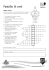WMO Task Force on Social and Economic Applications of Public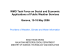spatio-temporal description of the rainfall in the andean city of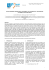View Presentation - University of the Philippines Diliman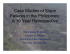Agroforestry - Indonesian Agency for Agricultural Research and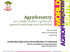A Cloud and Precipitation Feature Database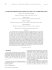Design of a rain rate retrieval algorithm using artificial neural